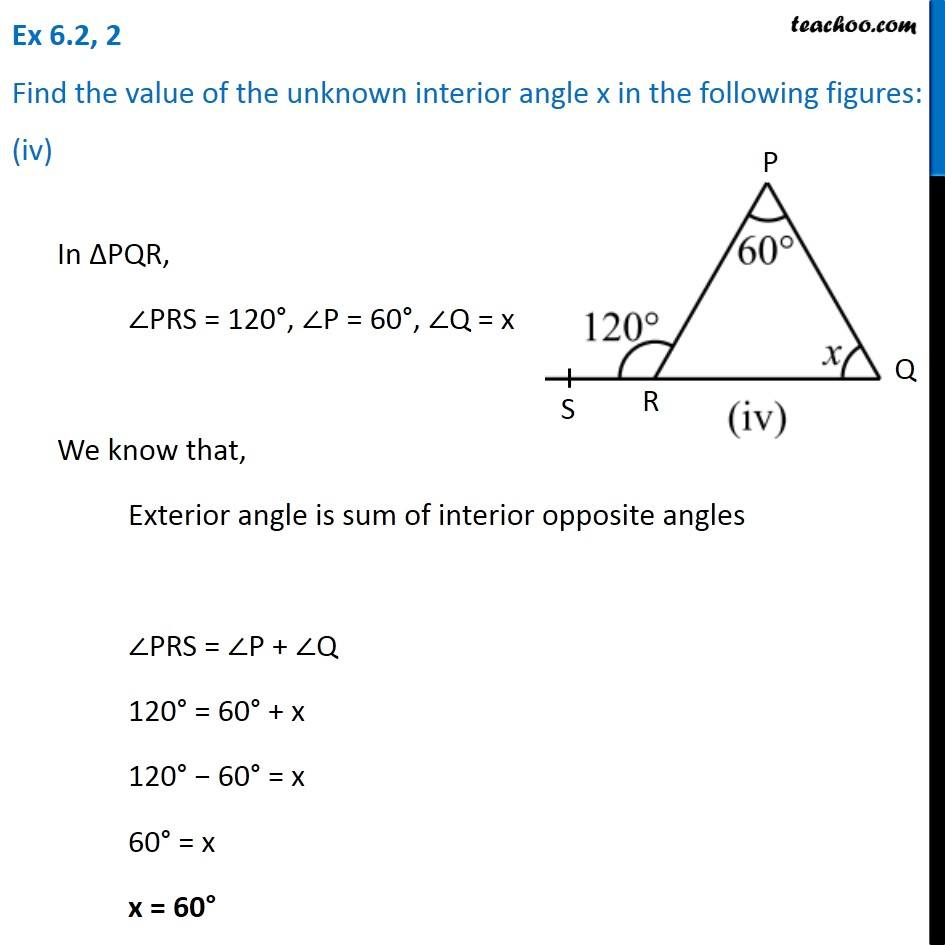Ex 6.2

Chapter 6 Class 7 Triangle and its Properties
Serial order wiseLearn in your speed, with individual attention - Teachoo Maths 1-on-1 Class

### Transcript

Ex 6.2, 2 Find the value of the unknown interior angle x in the following figures: (iv)In ∆PQR, ∠PRS = 120°, ∠P = 60°, ∠Q = x We know that, Exterior angle is sum of interior opposite angles ∠PRS = ∠P + ∠Q 120° = 60° + x 120° − 60° = x 60° = x x = 60°